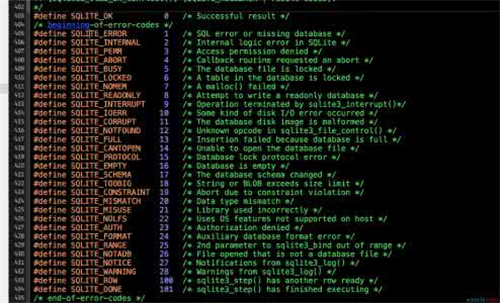• 主要介绍了C语言十进制转二进制代码实例,并且转换后会统计二进制1的个数,实例简单明了,需要的朋友可以参考下
• } if(data==0) printf("该十进制整数对应的二进制数为：\n0\n"); while(shang>0) { yushu=shang%2; p=(LinkList)malloc(sizeof(LNode)); if(!p) exit(-2); p->data=yushu; p->next=L->next; L->next=p; shang/=2; }...

该楼层疑似违规已被系统折叠 隐藏此楼查看此楼

//用栈结构

#include

#include

typedef struct LNode

{

int data;

struct LNode *next;

/*初始化*/

{

L->next=NULL;

return L;

}

/*转制*/

{

int sign=1,yushu,shang=data;

if(data<0)

{

shang=-data;

sign=-1;

}

if(data==0)

printf("该十进制整数对应的二进制数为：\n0\n");

while(shang>0)

{

yushu=shang%2;

if(!p)

exit(-2);

p->data=yushu;

p->next=L->next;

L->next=p;

shang/=2;

}

if(sign==-1)

{

printf("该十进制整数对应的二进制数为：\n");

printf("-");

}

for(p=L->next;p!=NULL;p=p->next)

printf("%d",p->data);

printf("\n");

}

/*销毁栈*/

{

while(!p)

{

q=p;

p=p->next;

free(q);

}

}

void main()

{

int data;

L=creatLNode(L);

printf("请输入要转换的十进制数：");

scanf("%d",&data);

zhuanzhi(L,data);

del(L);

}

展开全文•C
•c语言
• 十进制转二进制可以使用库函数itoa。itoa函数原型：char*itoa(int value,char*string,int radix);功能：将任意类型的数字转换为字符串。在中与之有相反功能的函数是atoi。nt value 被转换的整数，char *string 转换...

十进制转二进制可以使用库函数itoa。

功能：将任意类型的数字转换为字符串。在中与之有相反功能的函数是atoi。

nt value 被转换的整数，char *string 转换后储存的字符数组，int radix 转换进制数，如2,8,10,16 进制等。扩展资料:

方法

#include

int main(void)

{ int i=0,n,a;

printf("请输入一个十进制整数.\n");

scanf("%d",&n);

while (n>0)

{a[i]=n%2;

i=i+1;

n=n/2;  }

printf("十进制整数转换为二进制数是:\n");

for(i--;i>=0;i--)

printf("%d",a[i]);

printf("\n");

return 0;}

十进制整数转换为二进制整数的方法：

十进制整数转换为二进制整数采用"除2取余，逆序排列"法。

具体做法是：用2去除十进制整数，可以得到一个商和余数；再用2去除商，又会得到一个商和余数，如此进行，直到商为零时为止，然后把先得到的余数作为二进制数的低位有效位，后得到的余数作为二进制数的高位有效位，依次排列起。

展开全文• 问题描述：实现一个函数：能使十进制数转为二进制数代码实现：实际上可以利用C语言中的itoa函数 [itoa函数是C/C++的非标准函数，不保证被所有编译器正常使用]具体用法：char * itoa ( int value, char * str, int ...

问题描述：

实现一个函数：能使十进制数转为二进制数

代码实现：

实际上可以利用C语言中的itoa函数 [itoa函数是C/C++的非标准函数，不保证被所有编译器正常使用]

具体用法：char * itoa ( int value, char * str, int base );

value是待转换的十进制数，str用于存储转换后的字符串，base表示转换成多少进制

#include

#include

int main()

{

int a=30;

char str;

itoa(a,str,2);

printf("%s",str);

return 0;

}输出结果

除了用C语言自带函数外，也可以自己写函数，以下是我写的一个使用短除法的函数

void PositiveIntToBinary3(int value,int bits)

{

char binary[bits];

int index=0;

while(value)

{

if(index>=bits)

{

printf("error:over the limit!");

return;

}

value%2 ? binary[index++]='1' : binary[index++]='0';

value/=2;

}

//将剩余位数上的字符都置为‘0’

for(int i=index;i

binary[i]='0';

//倒序打印出字符数组

for(int i=bits-1;i>=0;i--)

printf("%c",binary[i]);

}

参数bits用于指定输出的二进制的最大长度，若value转化为二进制后长度大于bits，则会显示error:over the limit!

使用该函数：

int main()

{

PositiveIntToBinary3(246,16);

return 0;

}输出结果

代码思考：

打完代码后认真看一看这段代码还有什么可以简化的地方。

在上面的代码中，我发现：

//将剩余位数上的字符都置为‘0’

for(int i=index;i

binary[i]='0';

这个填充剩余字符的部分可以由已有的while循环实现。

改进版如下：

void PositiveIntToBinary4(int value,int bits)

{

char binary[bits];

int index=0;

while(index

{

value%2 ? binary[index++]='1' : binary[index++]='0';

value/=2;

}

if(value)

{

printf("error: over the limit!");

return;

}

for(int i=bits-1;i>=0;i--)

printf("%c",binary[i]);

}

我并不是什么聪慧的人，但我想写出好的代码。只有不断尝试和练习才能不断进步。如果你有更好的实现方法或者发现那些地方可以写的更加简洁高效的话，请告诉我，大家共同学习。

这是我在简书第一次写文章，希望这篇文章的内容能帮助您。本人水平有限也有请各位见谅。

展开全文• c语言十进制转换二进制 递归

千次阅读 多人点赞 2020-03-17 17:07:16
本题要求实现一个函数，将正整数n转换为二进制后输出。 函数接口定义： void dectobin( int n ); 函数dectobin应在一行中打印出二进制的n。建议用递归实现。 裁判测试程序样例： #include <stdio.h> void ...

本题要求实现一个函数，将正整数n转换为二进制后输出。

函数接口定义：

void dectobin( int n );

函数dectobin应在一行中打印出二进制的n。建议用递归实现。

裁判测试程序样例：

#include <stdio.h>

void dectobin( int n );

int main()
{
int n;

scanf("%d", &n);
dectobin(n);

return 0;
}

/* 你的代码将被嵌在这里 */

我的答案：

void dectobin(int n)
{
int result = 0;

if (n == 0)
{
printf("0");
}
else if (n == 1)
{
printf("1");
}
else
{
dectobin(n / 2);//这里用到了递归
result = n % 2;
printf("%d", result);
}
}

这里要注意对递归的理解
这里如果n没有对于0，1的话
会一直递归
直到n等于0或1才停止
最后输出是从较小的n向较大n顺序输出的

展开全文c语言
• 你赶上好运了，哈哈，我刚也做这个题，刚做好！求采纳哦 #include #include #define max 100 typedef struct { int a... } printf("转化进为："); while(!stackemply(s)) { pop(s,e); printf("%d",e); } return 0; }
• 借助栈的特性，十进制转二进制转换用递归写还蛮方便的 #include<stdio.h> void Dec2Bin(int num){ if(num==1) //num等于1 最后一项了 结束递归 { printf("%d",num); return; } Dec2Bin(num/2); ...c语言 递归法
• 将一个十进制正(负)整数转换为对应的二进制补码(用指针完成 十进制转二进制:1.先判断该整数是正数还是负数 如果是正数则二进制补码首位为1 , 且对应的二进制补码就是原. 如果是 负数 则二进制补码为原码基础上取反且...补码 c#
• //最后再二进制pwm_table return transform_data_zhengshu(sum,pwm_table); } //正数 return transform_data_zhengshu(frequence,pwm_table); } int main(){ int frequence = -2; //scanf(...C 进制转换
• C语言十进制转换二进制八，十六进制。十进制转二进制有个计算公式，就是除以2取余再倒序显示余数就是了。可以根据公式写。八制进公式也同理。十六进制有点不一样，大于9的要转为字母。A,B,C,D,E,F。#include void ...
• C语言十进制转换为二进制

千次阅读 2021-10-25 12:20:25
十进制如何转二进制：将该数字不断除以2直到商为零，然后将余数由下至上依次写出，即可得到该数字的二进制表示。 以将数字10转化为二进制为例 当商为零时，将余数由下至上依次写出，即为10的二进制表示 #include &...c语言 开发语言
• void fun25(void) {  int i, j, num;  unsigned ask = 0x8000; scanf("%d", &num); //方法一 for (i = 0;... //方法 while (num != 0) { printf("%d", num % 2); num /= 2; } }
• C语言实现十进制转换成八十六进制的转换，代码已经经过调试可以使用，放心下载！C语言
• #include void decimalToBinary(int n); void main() { int n; char again; do { printf("输入10进制："); scanf("%d",&n); printf("%d的二进制：",n); decimalToBinary(n);C语言十进制转二进制 C语言十进制转八进制
• #将10进制转为x进制 def change10_x ( n , x ) : string = '' while n != 0 : m = n % x string += str ( m ) n = int ( n / x ) return string [ : : - 1 ]
• #include <stdio.h> #include <math.h> void D_O(int n); void D_H(int n); void D_B(int n); void main() { int x,n; while (1) ... printf("输入十进制数:\n"); scanf_s("%d", &n.c语言 switch
• 这是一个C语言 do while 循环示例：统计十进制正整数 n 转换为二进制数后，其二进制序列中包含的 1 和 0 的个数。问题分析输入：一个正整数。输出：两个整数：1 的个数和 0 的个数。将十进制数 n 转换成二进制数，...
• c语言十进制转换成二进制 递归实现

千次阅读 多人点赞 2019-01-05 17:09:13
c语言 十进制转换成二进制 递归实现，函数代码很短，但是很难懂！ int fun(int n) //十进制转换成二进制递归函数 { if(n) //递归停止的条件 { fun(n/2); //递归 printf("%d",n&amp;1); } } ...c语言代码 递归 十进制转换
• C语言十进制转换为二进制C语言十进制转换为二进制完整源码 C语言十进制转换为二进制完整源码 #include <stdio.h> #include <stdlib.h> #define MAXBITS 100 int main() { // input of the user int ...
• #include<stdio.h> void main(){ ... printf("%d的二进制数为：",num); while(num!=0){ a[i]=num%2; num=num/2; i++; } for(i=i-1;i>=0;i--){ printf("%d",a[i]); } } ...c语言
• 把开发过程比较重要的一些内容备份一次，下边资料是关于C语言十进制转换成二进制的内容，应该对各位朋友有一些用。#include int main(){int num;std::cout << "Enter a number: ";std::cin >> num;std::...
• c语言二进制转换成十进制的方法发布时间：2020-04-09 09:52:38来源：亿速云阅读：648作者：小新今天小编给大家分享的是c语言二进制转换成十进制的方法，很多人都不太了解，今天小编为了让大家更加了解二进制转换成...
• 主要介绍了C语言用栈实现十进制转换为二进制的方法,结合实例形式分析了C语言栈的定义及进制转换使用技巧,需要的朋友可以参考下...

c语言十进制转二进制c语言 订阅